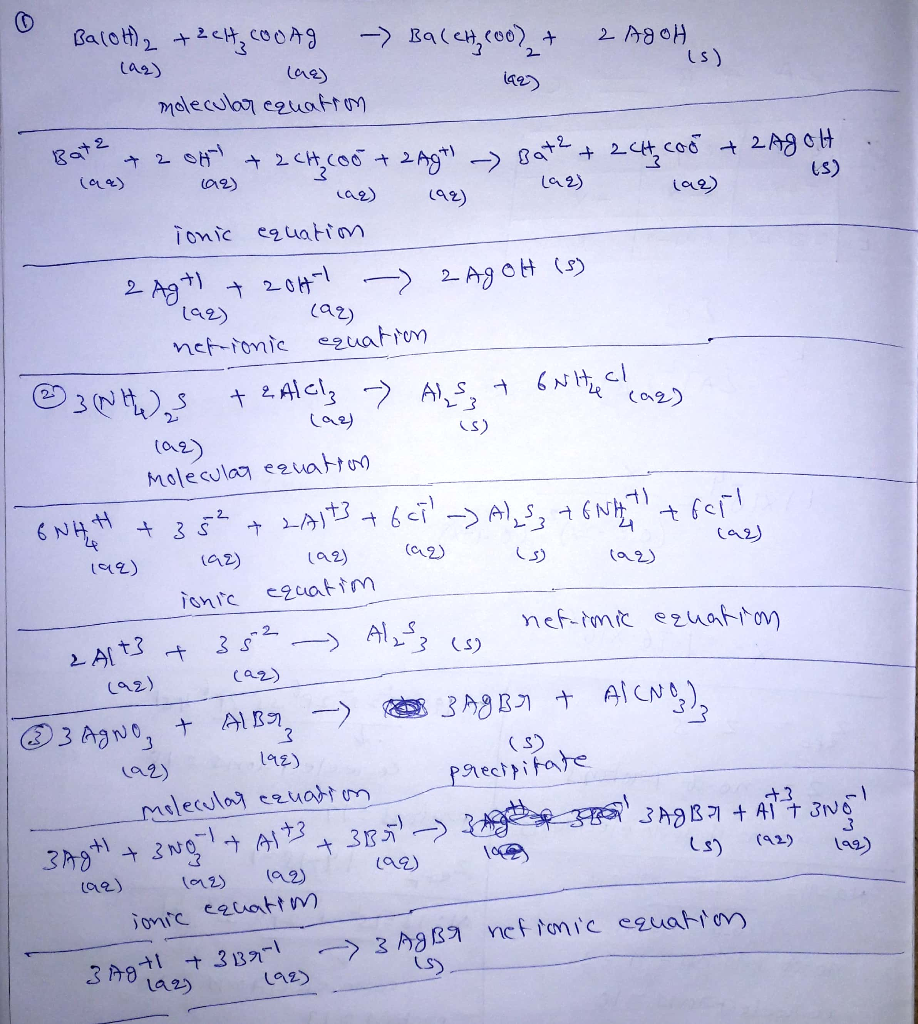In: Chemistry

# 1.Consider the reaction when aqueous solutions of barium hydroxide and silver(I) acetate are combined. The net...

1.Consider the reaction when aqueous solutions of barium hydroxide and silver(I) acetate are combined. The net ionic equation for this reaction is:

2.Consider the reaction when aqueous solutions of ammonium sulfide and aluminum chloride are combined. The net ionic equation for this reaction is:

3. A precipitate forms when aqueous solutions of silver(I) nitrate and aluminum bromide are combined.

4. A precipitate forms when aqueous solutions of silver(I) nitrate and aluminum bromide are combined.

## Solutions

##### Expert Solution## Related Solutions

##### 1.Consider the reaction when aqueous solutions of Chromium(II) iodide and sodium acetate are combined. The net...
1.Consider the reaction when aqueous solutions of Chromium(II) iodide and sodium acetate are combined. The net ionic equation for this reaction is 2.Consider the reaction when aqueous solutions of lead(II) acetate and manganese(II) iodide are combined. The net ionic equation for this is 3. Consider the reaction when aqueous solutions of lead(II) nitrate and manganese (II) bromide are combined. The net ionic equation for this is 4. Write the balanced net ionic equation for this reaction. Pb(NO3)2(aq)+MnI2(aq)->PbI2(s)+Mn(NO3)2(aq) 5. Write a...
##### 1A.Consider the reaction when aqueous solutions of nickel(II) sulfate and barium bromide are combined. The net...
1A.Consider the reaction when aqueous solutions of nickel(II) sulfate and barium bromide are combined. The net ionic equation for this reaction is: 1B.Consider the reaction when aqueous solutions of lead(II) acetate and potassium carbonate are combined. The net ionic equation for this reaction is: 1C.Consider the reaction when aqueous solutions of lead(II) nitrate and sodium hydroxide are combined. The net ionic equation for this reaction is: 2A.Write a net ionic equation for the reaction that occurs when aqueous solutions of...
##### 1.Write a balanced equation for the precipitation reaction that occurs when aqueous solutions of silver(I) nitrate...
1.Write a balanced equation for the precipitation reaction that occurs when aqueous solutions of silver(I) nitrate and sodium carbonate are combined. 2.Write the net ionic equation for the precipitation reaction that occurs when aqueous solutions of nickel(II) nitrate and potassium phosphate are combined. 3.Write a net ionic equation for the overall reaction that occurs when aqueous solutions of ascorbic acid (H2C6H6O6) and sodium hydroxide are combined. 4.Write a net ionic equation for the reaction that occurs when solid barium carbonate...
##### 1. Which of the following aqueous solutions are good buffer systems? . 0.10 M barium hydroxide...
1. Which of the following aqueous solutions are good buffer systems? . 0.10 M barium hydroxide + 0.23 M barium chloride 0.20 M nitric acid + 0.17 M sodium nitrate 0.20 M hypochlorous acid + 0.20 M sodium hypochlorite 0.26 M ammonia + 0.33 M ammonium bromide 0.38 M potassium nitrate + 0.26 M potassium bromide 2. Which of the following aqueous solutions are good buffer systems? . 0.26 M hydrobromic acid + 0.15 M sodium bromide. 0.35 M calcium...
##### 1.) Write a net ionic equation for the reaction that occurs when aqueous solutions of ammonia...
1.) Write a net ionic equation for the reaction that occurs when aqueous solutions of ammonia and hydrochloric acid are combined. 2.)Write a net ionic equation for the reaction that occurs when aqueous solutions of ammonia and nitrous acid are combined. 3.) Write a net ionic equation for the reaction that occurs when aqueous solutions of perchloric acid and ammonia are combined.
##### Write a net ionic equation for the reaction that occurs when aqueous solutions of ammonia and...
Write a net ionic equation for the reaction that occurs when aqueous solutions of ammonia and hydrobromic acid are combined. Write a net ionic equation for the reaction that occurs when aqueous solutions of ammonia and acetic acid are combined. Write a net ionic equation for the reaction that occurs when excess nitric acid (aq) and sodium carbonate (aq) are combined. Write a net ionic equation for the reaction that occurs when chromium(II) sulfide and excess hydroiodic acid are combined....
##### Silver(I) nitrate reacts with barium bromide to form silver(I) bromide and barium nitrate. If 73.5 g...
Silver(I) nitrate reacts with barium bromide to form silver(I) bromide and barium nitrate. If 73.5 g of silver(I) nitrate reacts with 92.6 g of barium bromide, how many g of barium nitrate will be formed? How many g of the excess reactant will be left after the reaction occurs? How many g of silver(I) bromide will be formed? Do your calculations verify the Law of Conservation of Matter?
##### Silver(I) nitrate reacts with barium bromide to form silver(I) bromide and barium nitrate. If 73.5 g...
Silver(I) nitrate reacts with barium bromide to form silver(I) bromide and barium nitrate. If 73.5 g of silver(I) nitrate reacts with 92.6 g of barium bromide, how many g of barium nitrate will be formed? How many g of the excess reactant will be left after the reaction occurs? How many g of silver(I) bromide will be formed? Do your calculations verify the Law of Conservation of Matter?
##### The net ionic equation for the reaction between aqueous solutions of HF and KOH is.... a)...
The net ionic equation for the reaction between aqueous solutions of HF and KOH is.... a) HF + KOH ---> H2O + K^+ + F^- b) HF + OH^- ---> H2O + F^- c) HF + K^+ ----> H2O + KF d) H^+ + OH^- ----> H2O e) H^+ + F^- + K^+ + OH^- ---> H2O + K^+ + F^- can you please explain how you got to the answer, thank you!
##### When aqueous solutions of magnesium sulfate and potassium phosphate are combined, solid magnesium phosphate and a...
When aqueous solutions of magnesium sulfate and potassium phosphate are combined, solid magnesium phosphate and a solution of potassium sulfate are formed. The net ionic equation for this reaction is: (Specify states such as (aq) or (s)